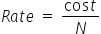Mathematics
Easy

Question

# A restaurant sells 4 boxes of chicken nuggets for \$18. The cost of 10 boxes of chicken nuggets will be ________________.

## 30354045Hint:

## The correct answer is: 45

### Cost of 4 boxes of chicken nuggets is \$18We have to find the rate. We will find it by taking the ratio of the cost of the boxes and number of boxes. As the rate is constant, we will use this value to find cost of 10 boxes.Let the number of boxes of chicken nuggets be denoted as ‘N’.So the equation becomeSo the rate = \$4.5 per bagLet’s find the cost of 10 boxesWe will rearrange this for cost.For this we will multiply both sides by ‘N’.Rate ×N = costCost = Rate × NSubstituting the values we have found.Cost = 4.5 × 10Cost = \$45Therefore, cost of 10 boxes of chicken nuggets is \$45.

We should be careful about the units. Same quantities must have same units before operation.

### Related Questions to study#### With Turito Foundation.#### Get an Expert Advice From Turito.